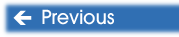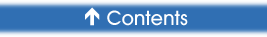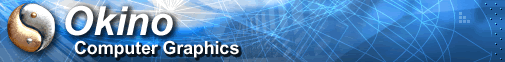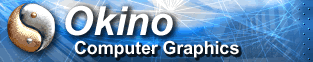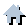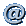You are here:   Home »  Specialized Sections#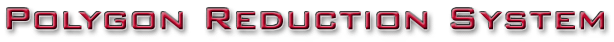A Quick Overview of the Okino Polygon Reduction System

Okino's polygon reduction algorithm is based on the technique described by Michael Garland in his Ph.D. thesis, Quadric-Based Polygonal Surface Simplification.

This technique uses what Garland calls quadric error metrics (QEM) and vertex pair contraction, which has been restricted to edge contraction in this application.

Edge contraction simply means that the two end vertices of a model edge are replaced by a single new vertex. This target vertex is usually somewhere in between the other two, in a place where it best approximates the original model. This edge contraction step removes a vertex and one, two, or more faces from the model, depending on the mesh neighborhood.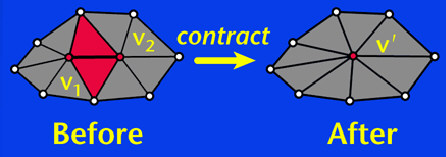When this step is repeated several times, it results in a simpler model, which is an approximation to the original. Since any time data is removed from the original model the resulting model will only be an approximation; each of these steps is associated with a given increase in error. Garland uses quadric error matrices to estimate the error of each possible edge being contracted, and stores the edge contractions in a heap in order of increasing error (also called the cost of contraction). At each step the contraction with the lowest error (at the top of the heap) is performed, and the errors associated with the affected edges have to be recalculated. Then the lowest cost contraction is performed again until the desired target face count is reached. Simplification can also be stopped when the lowest error contraction is above a certain error threshold.

(Image shown above, Copyright © Michael Garland. From a PDF slide presentation titled "Surface Simplification using Quadric Error Metrics". Michael Garland and Paul S. Heckbert. 1997. Carnegie Mellon).